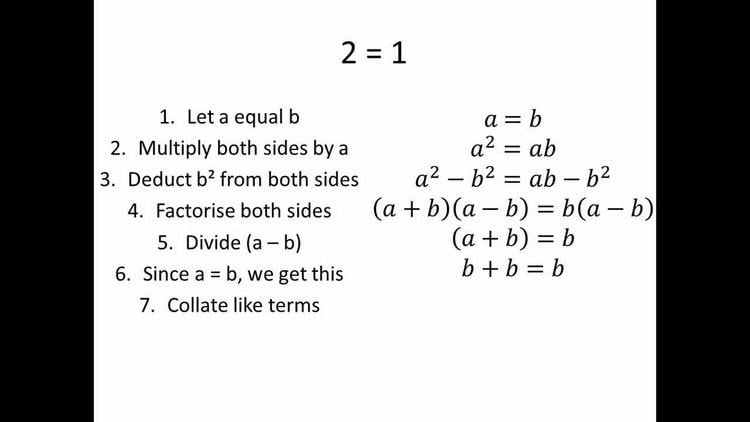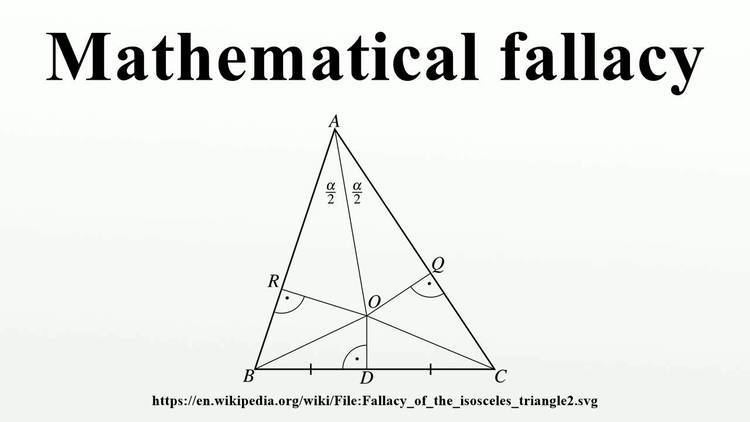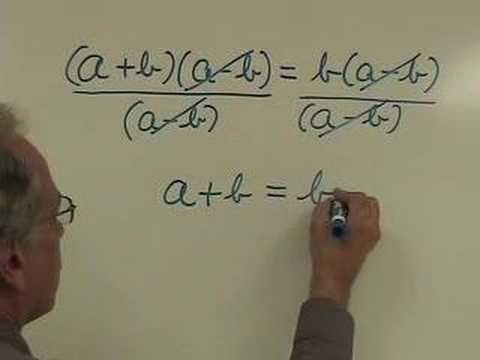# Mathematical fallacy

Updated on
Covid-19## A mathematical fallacy

In mathematics, certain kinds of mistaken proof are often exhibited, and sometimes collected, as illustrations of a concept of mathematical fallacy. There is a distinction between a simple mistake and a mathematical fallacy in a proof: a mistake in a proof leads to an invalid proof just in the same way, but in the best-known examples of mathematical fallacies, there is some concealment in the presentation of the proof. For example, the reason validity fails may be a division by zero that is hidden by algebraic notation. There is a striking quality of the mathematical fallacy: as typically presented, it leads not only to an absurd result, but does so in a crafty or clever way. Therefore, these fallacies, for pedagogic reasons, usually take the form of spurious proofs of obvious contradictions. Although the proofs are flawed, the errors, usually by design, are comparatively subtle, or designed to show that certain steps are conditional, and should not be applied in the cases that are the exceptions to the rules.

## ContentsThe traditional way of presenting a mathematical fallacy is to give an invalid step of deduction mixed in with valid steps, so that the meaning of fallacy is here slightly different from the logical fallacy. The latter applies normally to a form of argument that is not a genuine rule of logic, where the problematic mathematical step is typically a correct rule applied with a tacit wrong assumption. Beyond pedagogy, the resolution of a fallacy can lead to deeper insights into a subject (such as the introduction of Pasch's axiom of Euclidean geometry and the five colour theorem of graph theory). Pseudaria, an ancient lost book of false proofs, is attributed to Euclid.Mathematical fallacies exist in many branches of mathematics. In elementary algebra, typical examples may involve a step where division by zero is performed, where a root is incorrectly extracted or, more generally, where different values of a multiple valued function are equated. Well-known fallacies also exist in elementary Euclidean geometry and calculus.## Howlers

Examples exist of mathematically correct results derived by incorrect lines of reasoning. Such an argument, however true the conclusion, is mathematically invalid and is commonly known as a howler. Consider for instance the calculation (anomalous cancellation):

16 64 = 16 / 6 / 4 = 1 4 .

Although the conclusion 16 64 = 1 4 is correct, there is a fallacious, invalid cancellation in the middle step. Another classical example of a howler is proving the Cayley-Hamilton theorem by simply substituting the scalar variables of the characteristic polynomial by the matrix.

Bogus proofs, calculations, or derivations constructed to produce a correct result in spite of incorrect logic or operations were termed howlers by Maxwell. Outside the field of mathematics the term "howler" has various meanings, generally less specific.

## Division by zero

The division-by-zero fallacy has many variants. The following example uses division by zero to "prove" that 2 = 1 , but can be modified to prove that any number equals any other number.

1. Let a and b be equal, non-zero quantities a = b
2. Multiply by a a 2 = a b
3. Subtract b 2 a 2 b 2 = a b b 2
4. Factor both sides: the left factors as a difference of squares, the right is factored by extracting b from both terms ( a b ) ( a + b ) = b ( a b )
5. Divide out ( a b ) a + b = b
6. Observing that a = b b + b = b
7. Combine like terms on the left
8. Divide by the non-zero b 2 = 1

Q.E.D.

The fallacy is in line 5: the progression from line 4 to line 5 involves division by ab, which is zero since a equals b. Since division by zero is undefined, the argument is invalid.

## Multivalued functions

Many functions do not have a unique inverse. For instance squaring a number gives a unique value, but there are two possible square roots of a positive number. The square root is multivalued. One value can be chosen by convention as the principal value, in the case of the square root the non-negative value is the principal value, but there is no guarantee that the square root function given by this principal value of the square of a number will be equal to the original number, e.g. the square root of the square of −2 is 2.

## Calculus

Calculus as the mathematical study of infinitesimal change and limits can lead to mathematical fallacies if the properties of integrals and differentials are ignored. For instance, a naive use of integration by parts can be used to give a false proof that 0 = 1. Letting u = 1 log x and d v = d x x , we may write:

1 x log x d x = 1 + 1 x log x d x

after which the antiderivatives may be cancelled yielding 0 = 1. The problem is that antiderivatives are only defined up to a constant and shifting them by 1 or indeed any number is allowed. The error really comes to light when we introduce arbitrary integration limits a and b.

a b 1 x log x d x = 1 | a b + a b 1 x log x d x = 0 + a b 1 x log x d x = a b 1 x log x d x

Since the difference between two values of a constant function vanishes, the same definite integral appears on both sides of the equation.

## Power and root

Fallacies involving disregarding the rules of elementary arithmetic through an incorrect manipulation of the radical.

## Positive and negative roots

Care must be taken when taking the square root of both sides of an equality. Failing to do so results in a proof of

5 = 4.

Proof:

Start from Write this as Rewrite as Add 81 / 4 on both sides: 5 2 5 × 9 + 81 / 4 = 4 2 4 × 9 + 81 / 4 These are perfect squares: Take the square root of both sides: Add 9 / 2 on both sides: 5 = 4 Q.E.D.

The fallacy is in the last but one line, where the square root of both sides is taken: a2 = b2 only implies a = b if a and b have the same sign, which is not the case here. In this case it implies a = –b and should read 5-9/2 = -(4-9/2), which, by adding 9/2 on both sides, correctly reduces to 5 = 5.

As another example of the danger of squaring both sides of an equation, consider the fundamental identity

cos 2 x = 1 sin 2 x

which holds as a consequence of the Pythagorean theorem. Then, by taking a square root,

cos x = 1 sin 2 x

so that

1 + cos x = 1 + 1 sin 2 x .

But evaluating this when x = π implies

1 1 = 1 + 1 0

or

0 = 2

which is incorrect.

The error in each of these examples fundamentally lies in the fact that any equation of the form

x 2 = a 2

has two solutions, provided a ≠ 0,

x = ± a

and it is essential to check which of these solutions is relevant to the problem at hand. In the above fallacy, the square root that allowed the second equation to be deduced from the first is valid only when cos x is positive. In particular, when x is set to π, the second equation is rendered invalid.

## Squaring both sides of an equation

When both sides of an equation are squared, sometimes solutions are induced that were not present in the original equation.

An example of this kind of fallacy, is the following invalid proof that 2 = 2 :

Let Squaring gives whereupon taking a square root implies so that which is absurd.

Clearly when the square root was extracted, it was the negative root −2, rather than the positive root, that was relevant for the particular solution in the problem.

## Square roots of negative numbers

Invalid proofs utilizing powers and roots are often of the following kind:

1 = 1 = ( 1 ) ( 1 ) = 1 1 = i i = 1.

The fallacy is that the rule x y = x y is generally valid only if both x and y are positive (when dealing with real numbers), which is not the case here.

Alternatively, imaginary roots are obfuscated in the following:

i = 1 = ( 1 ) 2 4 = ( ( 1 ) 2 ) 1 4 = 1 1 4 = 1

The error here lies in the last equality, where we are ignoring the other fourth roots of 1, which are −1, i and −i (where i is the imaginary unit). Seeing as we have squared our figure and then taken roots, we cannot always assume that all the roots will be correct. So the correct fourth roots are i and −i, which are the imaginary numbers defined to square to −1.

## Complex exponents

When a number is raised to a complex power, the result is not uniquely defined (see Failure of power and logarithm identities). If this property is not recognized, then errors such as the following can result:

e 2 π i = 1 ( e 2 π i ) i = 1 i e 2 π = 1

The error here is that the rule of multiplying exponents as when going to the third line does not apply unmodified with complex exponents, even if when putting both sides to the power i only the principal value is chosen. When treated as multivalued functions, both sides produce the same set of values, being {e2πn | n ∈ ℤ}.

## Geometry

Many mathematical fallacies in geometry arise from using in an additive equality involving oriented quantities (such adding vectors along a given line or adding oriented angles in the plane) a valid identity, but which fixes only the absolute value of (one of) these quantities. This quantity is then incorporated into the equation with the wrong orientation, so as to produce an absurd conclusion. This wrong orientation is usually suggested implicitly by supplying an imprecise diagram of the situation, where relative positions of points or lines are chosen in a way that is actually impossible under the hypotheses of the argument, but non-obviously so. Such a fallacy is easy to expose by drawing a precise picture of the situation, in which some relative positions will be different from those in the provided diagram. In order to avoid such fallacies, a correct geometric argument using addition or subtraction of distances or angles should always prove that quantities are being incorporated with their correct orientation.

## Fallacy of the isosceles triangle

The fallacy of the isosceles triangle, from (Maxwell 1959, Chapter II, § 1), purports to show that every triangle is isosceles, meaning that two sides of the triangle are congruent. This fallacy has been attributed to Lewis Carroll.

Given a triangle △ABC, prove that AB = AC:

1. Draw a line bisecting ∠A
2. Draw the perpendicular bisector of segment BC, which bisects BC at a point D
3. Let these two lines meet at a point O.
4. Draw line OR perpendicular to AB, line OQ perpendicular to AC
5. Draw lines OB and OC
6. By AAS, △RAO ≅ △QAO (∠ORA = ∠OQA = 90; ∠RAO = ∠QAO; AO = AO (common side))
7. By RHS, △ROB ≅ △QOC
8. Thus, AR = AQ, RB = QC, and AB = AR + RB = AQ + QC = AC

Q.E.D.

As a corollary, one can show that all triangles are equilateral, by showing that AB = BC and AC = BC in the same way.

The error in the proof is the assumption in the diagram that the point O is inside the triangle. In fact, O always lies at the circumcircle of the △ABC (except for isosceles and equilateral triangles where AO and OD coincides . Furthermore, it can be shown that, if AB is longer than AC, then R will lie within AB, while Q will lie outside of AC (and vice versa). (Any diagram drawn with sufficiently accurate instruments will verify the above two facts.) Because of this, AB is still AR + RB, but AC is actually AQ − QC; and thus the lengths are not necessarily the same.

## Proof by induction

There exist several fallacious proofs by induction in which one of the components, basis case or inductive step, is incorrect. Intuitively, proofs by induction work by arguing that, if a statement is true in one case, it is true in the next case, and hence by repeatedly applying this it can be shown to be true for all cases. This "proof" shows that all horses are the same colour.

1. Let us say that any group of N horses is all of the same colour.
2. If we remove a horse from the group, we have a group of N - 1 horses of the same colour. If we add another horse, we have another group of N horses. By our previous assumption, all the horses are of the same colour in this new group, since it is a group of N horses.
3. Thus we have constructed two groups of N horses all of the same colour, with N - 1 horses in common. Since these two groups have some horses in common, the two groups must be of the same colour as each other.
4. Therefore, combining all the horses used, we have a group of N + 1 horses of the same colour.
5. Thus if any N horses are all the same colour, any N + 1 horses are the same colour.
6. This is clearly true for N = 1 (i.e. one horse is a group where all the horses are the same colour). Thus, by induction, N horses are the same colour for any positive integer N. i.e. all horses are the same colour.

The fallacy in this proof arises in line 3. For N = 1, the two groups of horses have N − 1 = 0 horses in common, and thus are not necessarily the same colour as each other, so the group of N + 1 = 2 horses is not necessarily all of the same colour. The implication "Every N horses are of the same colour, then N+1 horses are of the same colour" works for any N greater than one, but fails to be true when N = 1. The basis case is correct, but the induction step has a fundamental flaw. If we were additionally given the fact that any two horses shared the same colour, we could correctly induct from the base case of N = 2.

## References

Similar Topics
Oliver Bjorkstrand
Romeo Crennel
Kathy Galloway
Topics